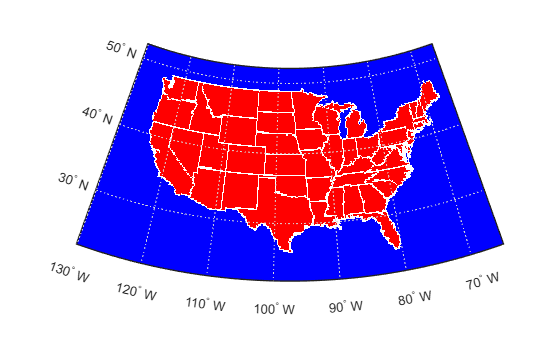# vec2mtx

Convert latitude-longitude vectors to regular data grid

## Syntax

```[Z,R] = vec2mtx(lat,lon,density) [Z,R] = vec2mtx(lat,lon,density,latlim,lonlim) [Z,R] = vec2mtx(lat,lon,Z1,R1) [Z,R] = vec2mtx(...,'filled') ```

## Description

`[Z,R] = vec2mtx(lat,lon,density)` creates a regular data grid `Z` from vector data, placing ones in grid cells intersected by a vector and zeroes elsewhere. `R` is the raster reference object for the computed grid. `lat` and `lon` are vectors of equal length containing geographic locations in units of degrees. `density` indicates the number of grid cells per unit of latitude and longitude (a value of 10 indicates 10 cells per degree, for example), and must be scalar-valued. Whenever there is space, a buffer of two grid cells is included on each of the four sides of the grid. The buffer is reduced as needed to keep the latitudinal limits within [-90 90] and to keep the difference in longitude limits from exceeding 360 degrees.

`[Z,R] = vec2mtx(lat,lon,density,latlim,lonlim)` uses the two-element vectors `latlim` and `lonlim` to define the latitude and longitude limits of the grid.

`[Z,R] = vec2mtx(lat,lon,Z1,R1)` uses a pre-existing data grid `Z1`, georeferenced by `R1`, to define the limits and density of the output grid. `R1` can be a referencing vector, a referencing matrix, or a geographic raster reference object.

If `R1` is a geographic raster reference object, its `RasterSize` property must be consistent with `size(Z1)` and its `RasterInterpretation` must be `'cells'`.

If `R1` is a referencing vector, it must be a 1-by-3 vector containing these elements:

`[cells/degree northern_latitude_limit western_longitude_limit]`

If `R1` is a referencing matrix, it must be 3-by-2 and transform raster row and column indices to or from geographic coordinates according to this equation:

`[lon lat] = [row col 1] * R1`

The matrix must define a (non-rotational, non-skewed) relationship in which each column of the data grid falls along a meridian and each row falls along a parallel.

With this syntax, the output `R` is equal to `R1`, and may be a referencing object, vector, or matrix.

`[Z,R] = vec2mtx(...,'filled')`, where `lat` and `lon` form one or more closed polygons (with `NaN`-separators), fills the area outside the polygons with the value two instead of the value zero.

## Notes

Empty `lat,lon` vertex arrays will result in an error unless the grid limits are explicitly provided (via `latlim,lonlim` or `Z1,R1`). In the case of explicit limits, `Z` will be filled entirely with 0s if the `'filled'` parameter is omitted, and 2s if it is included.

It's possible to apply `vec2mtx` to sets of polygons that tile without overlap to cover an area, as in Example 1 below, but using `'filled'` with polygons that actually overlap may lead to confusion as to which areas are inside and which are outside.

## Examples

collapse all

Convert latitude-longitude polygons to a regular data grid and display as a map.

```states = shaperead('usastatelo', 'UseGeoCoords', true); lat = [states.Lat]; lon = [states.Lon]; [Z,R] = vec2mtx(lat,lon,5,'filled'); figure worldmap(Z,R) geoshow(Z,R,'DisplayType','texturemap') colormap(flag(3))```Combine two separate calls to `vec2mtx` to create a 4-color raster map showing interior land areas, coastlines, oceans, and world rivers.

```load coastlines [Z,R] = vec2mtx(coastlat,coastlon,1, ... [-90 90],[-90 270],'filled'); rivers = shaperead('worldrivers.shp','UseGeoCoords',true); A = vec2mtx([rivers.Lat],[rivers.Lon],Z,R); Z(A == 1) = 3; figure worldmap(Z,R) geoshow(Z,R,'DisplayType','texturemap') colormap([.45 .60 .30; 0 0 0; 0 0.5 1; 0 0 1])```Import US state polygons as a geospatial table. Extract the coordinates of the polygons by converting the geospatial table to a table.

```states = readgeotable("usastatelo.shp"); T = geotable2table(states,["Latitude" "Longitude"]); [lat,lon] = polyjoin(T.Latitude',T.Longitude');```

Choose geographic limits.

```latlim = [ 15 75]; lonlim = [-190 -65];```

Specify a grid with 5 cells per degree.

`density = 5;`

Compute raster size. (M and N both work out to be integers.)

```M = density * diff(latlim); N = density * diff(lonlim);```

Create a geographic raster reference object.

```R = georasterref("RasterSize", [M N], ... "ColumnsStartFrom","north","Latlim",latlim, ... "Lonlim",lonlim);```

Create a blank grid that is consistent with `R` in size. `vec2mtx` requires a data grid as input.

`Z = zeros(R.RasterSize);`

Overwrite `Z` with a new grid including state outlines and interiors.

`Z = vec2mtx(lat,lon,Z,R,"filled");`

Plot the georeferenced grid.

```figure; worldmap(Z,R); geoshow(Z,R,"DisplayType", "texturemap") colormap(flag(3))```## Version History

Introduced before R2006a

expand all

Behavior changed in R2021a